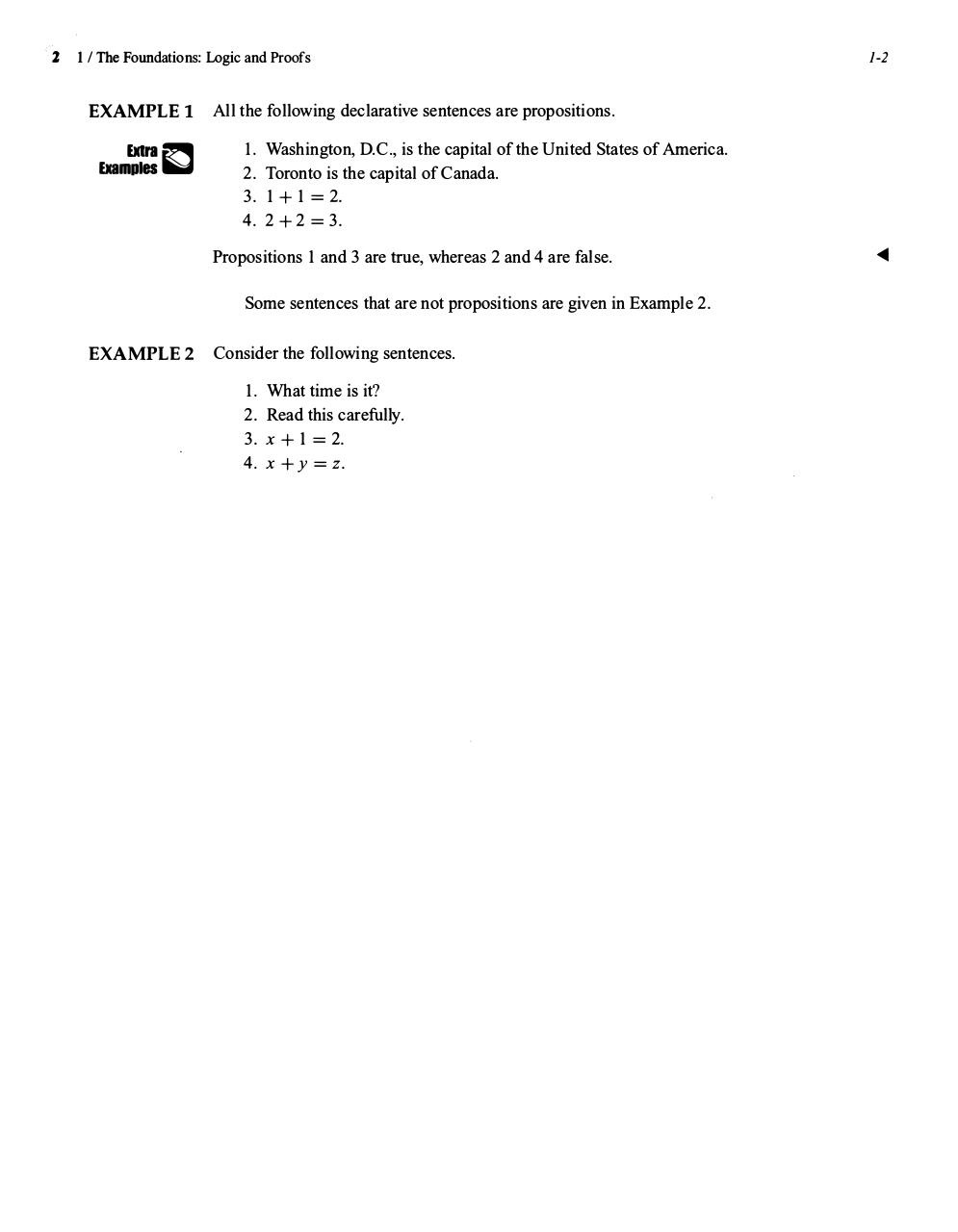# 1+&+2.pdfPage 12322

#### Text preview

2

1 -2

1 / The Foundations: Logic and Proofs

EXAMPLE l

Exam= �

All the following declarative sentences are propositions.

1 . Washington, D.C., is the capital of the United States of America.
2 . Toronto is the capital of Canada.
3. 1 + 1 = 2 .
4. 2 + 2 = 3 .
Propositions

1

and

3

are true, whereas

2

and 4 are false.

Some sentences that are not propositions are given in Example

EXAMPLE 2

Consider the following sentences.

1.
2.
3.
4.

What time is it?